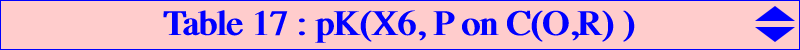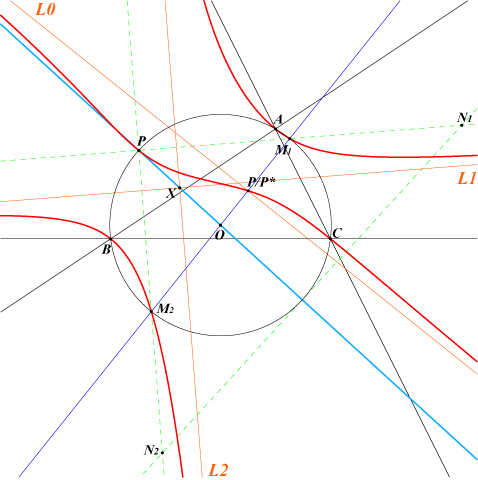Let P be a point on the circumcircle and P* its isogonal conjugate at infinity. Any pK(X6, P) can be seen as the locus of anallagmaty centers of the isogonal focal nKs with singular focus P. See CL027. This pK is unusual since it is possible to draw its three (always real) asymptotes with ruler and compasses only which is in general not possible (see Special Isocubics §1.4.4). Indeed, the pK meets the circumcircle at P and two other points M1, M2 lying on the perpendicular at O to the line PP* or equivalently, the parallel at O to the Simson line S(P) of P. Obviously, P*, M1* and M2* are the three points at infinity. This shows that the pK has two perpendicular asymptotes L1, L2 passing through M1*, M2* and another one L0 passing through P*. The isogonal transform of the pK with respect to the triangle PM1M2 is a central cubic with center O and whose asymptotes are the perpendicula bisectors of ABC. See CL065 for further properties and a generalization. Construction of L0 The tangential of P* is P/P* (cevian quotient) hence L0 contains this point. The perpendiculars at A, B, C to S(P) meet BC, CA, AB at A1, B1, C1. The perspector of the cevian triangle of P and the triangle formed by the midpoints of AA1, BB1, CC1 is P/P*. Hence, L0 is the perpendicular at this point to S(P). Remember that the tangents at A, B, C, P to the pK are also perpendicular to S(P). Construction of L1 and L2 Denote by N1, N2 the reflections of P in M1, M2 and by N1*, N2* their isogonal conjugates. L1, L2 are the parallels at N1*, N2* to the lines PM1, PM2. L1, L2 intersect at X on the line OP. See the figure below. The following table gives a selection of such pKs. Note that the cubic contains a given point Q (and its isogonal conjugate Q*) if and only if its pivot is one (if any) of the two intersections of the line QQ* and the circumcircle.P pK(X6, P) centers X(i) on the cubic for i = X(98) 1, 98, 511, 1687, 1688, 1756, 2009, 2010 X(99) 1, 39, 83, 99, 512, 1018, 1019, 1379, 1380 X(100) 1, 100, 513, 1381, 1382 X(101) 1, 101, 514, 2140, 2141 X(104) 1, 36, 80, 104, 517 X(105) 1, 105, 238, 291, 518 X(110) 1, 5, 54, 110, 523, 1113, 1114, 2574, 2575 X(476) 1, 30, 74, 110, 476, 523, 526 X(733) 1, 39, 83, 732, 733 X(805) 1, 98, 99, 511, 512, 804, 805 X(813) 1, 238, 291, 812, 813, 1018, 1019 X(901) 1, 100, 104, 513, 517, 900, 901 X(927) 1, 101, 103, 514, 516, 926, 927 X(932) 1, 667, 668, 932 X(934) 1, 905, 934, 1783 X(1113) 1, 3, 4, 1113, 2574 X(1114) 1, 3, 4, 1114, 2575 X(1141) 1, 5, 54, 1141, 1154, 1157, 1263 X(1291) 1, 1157, 1263, 1291 X(1309) 1, 102, 109, 515, 522, 1309 X(2222) 1, 36, 80, 2222